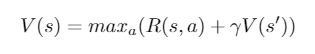02. Reinforcement Learning (Move 37): The Bellman Equation

A summary of the concepts discussed in the second lecture for a reinforcement learning course from the School of AI called Move 37. The focus of this lecture was on one variation of the Bellman Equation. See my previous post here for the introductory lecture notes summary.

Review of Concepts

State: a numeric representation of what the agent is observing at a particular point of time in the environment

Action: the input the agent provides to the environment, calculated by applying a policy to a current state.

Reward: a feedback signal from the environment reflecting how well the agent is performing the goals of the game

Goal of Reinforcement Learning

Given the current state we are in, choose the optimal action which will maximise the long-term expected reward provided by the environment.

Dynamic Programming

Reinforcement learning is based on Dynamic Programming.

Dynamic Programming is a class of algorithms, which seek to simplify complex problems by breaking them up into sub-problems and solving the sub-problems recursively (by a function that calls itself)

What question does the Bellman Equation answer?

• Given the state I’m in, assuming I take the best possible action now and at each subsequent step, what long-term reward can I expect?
• What is the VALUE of the STATE?
• Helps us evaluate the expected reward relative to the advantage or disadvantage of each state

Bellman Equation (for deterministic environments)

The value of a given state is equal to the max action (action which maximises the value) of the reward of the optimal action in the given state and add discount factor (diminishes the reward over time) multiplied by the next state’s Value from the Bellman Equation.

How to find the best action (maxₐ)?

• Brute force
• Deep neural nets

Importance of the discount factor (γ):

• It is important to tune this hyperparameter to get optimum results
• Successful values range between 0.9 and 0.99
• A lower value encourages short-term thinking
• A higher value emphasises long-term rewards

Side Note: The equation is recursive so it will start from the max reward and calculate backwards.

Since the lecture video for the course was private, I found a good alternative video explanation of the application of the Bellman Equation highlighted above.

The next summary will contain further details of the Markov Decision Processes. If you would like post updates follow me here and/or on Twitter.

Source: Deep Learning on Medium